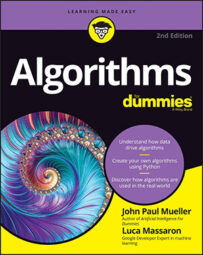##### Algorithms For DummiesWhen faced with a new difficult problem, it's not hard to come up with a greedy solution using the four steps described in the previous section. All you have to do is divide your problems into phases and determine which greedy rule to apply at each step. That is, you do the following:
• Choose how to make your decision (determine which approach is the simplest, most intuitive, smallest, and fastest)
• Start solving the problem by applying your decision rule
• Record the result of your decision (if needed) and determine the status of your problem
• Repeatedly apply the same approach at every step until reaching the problem conclusion
No matter how you apply the previous steps, you must determine whether you're accomplishing your goal by relying on a series of myopic decisions. The greedy approach works for some problems and sometimes for specific cases of some problems, but it doesn't work for every problem. For instance, the make-change problem works perfectly with U.S. currency but produces less-than-optimal results with other currencies. For example, using a fictional currency (call it credits, using a term in many sci-fi games and fiction) with denominations of 1, 15, and 25 credits, the previous algorithm fails to deliver the optimal change for a due sum of 30 credits:

```print ('Change: %s (using %i bills)' ``` ```% (change(30, [25, 15, 1]))) ``` ```Change: [25, 1, 1, 1, 1, 1] (using 6 bills) ``` Clearly, the optimal solution is to return two 15 credit bills, but the algorithm, being shortsighted, started with the highest denomination available (25 credits) and then used five 1 credit bills to make up the residual 5 credits.

Some complex mathematical frameworks called matroids can help verify whether you can use a greedy solution to optimally solve a particular problem. If phrasing a problem using a matroid framework is possible, a greedy solution will provide an optimal result. Yet there are problems that have optimal greedy solutions that don't abide by the matroid framework. (You can read about matroid structures being sufficient, but not necessary for an optimal greedy solution.)

The greedy algorithms user should know that greedy algorithms do perform well but don't always provide the best possible results. When they do, it's because the problem consists of known examples or because the problem is compatible with matroid mathematical framework. Even when a greedy algorithm works best in one setting, changing the setting may break the toy and generate just good or acceptable solutions. In fact, the cases of just good or acceptable results are many, because greedy algorithms don't often outperform other solutions, as shown by
• The make-change problem solutions show how a change in setting can cause a greedy algorithm to stop working.
• The scheduling problem illustrates how a greedy solution works perfectly with one worker, but don't expect it to work with more than one.
• The Dijkstra shortest-path algorithm works only with edges having positive weights. (Negative weights will cause the algorithm to loop around some nodes indefinitely.)
Demonstrating that a greedy algorithm works the best is a hard task, requiring a solid knowledge of mathematics. Otherwise, you can devise a proof in a more empirical way by testing the greedy solution against one of the following:
• Against a known optimal solution, when the greedy algorithm produces the optimal solution or you can change such a solution by exchanging its elements into an equivalent best solution (without any loss of performance or success). When a greedy solution matches the result of an optimal solution, you know that the greedy solution is equivalent and that it works best (this is the exchange proof).
• Against another algorithm when, as you see the greedy solution unfolding, you notice that the greedy solution stays ahead of the other algorithm; that is, the greedy solution always provides a better solution at every step than is provided by another algorithm.

Even considering that it's more the exception than a rule that a successful greedy approach will determine the top solution, greedy solutions often outperform other tentative solutions. You may not always get the top solution, but the solution will provide results that are good enough to act as a starting point (as a minimum), which is why you should start by trying greedy solutions first on new problems.